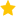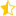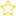See the live dashboard for CensusAtSchool 2023

We continue exploring trends, extracting seasonal patterns and forecasting for time series data. How big is the seasonal effect? We’ll discuss two models for decomposing a basic time series plot by separating out the trend, seasonal effect and residuals. Once we have learned how to decompose a time series plot using an additive model, we’ll look at another type of model, the multiplicative model. We’ll learn to compare the characteristics of the two types of models and decide which one would be a better fit to our data. Finally, we can use the best model to make predictions about the future.

The movie has been split into two approximately 5-minute parts. (See also the Review Questions following these two movies.)

[Illustrated transcript (pdf) for Part I]

[Illustrated transcript (pdf) for Part II]

After you’ve watched these videos, you should be able to answer these questions:

• What is the basic idea behind an additive model (or additive seasonal decomposition)?
• What is the basic idea behind a multiplicative model (or multiplicative seasonal decomposition)?
• Why do we want to find stable structures in our time series?
• When is an additive model likely to work better and when is a multiplicative model likely to work better?
• When we have an additive decomposition, how do we adjust the trend value to incorporate the seasonal effect at each time point?
• When we have a multiplicative decomposition, how do we adjust the trend value to incorporate the seasonal effect at each time point?
• What is the no-change value when we are making additive adjustments?
• What is the no-change value when we are making multiplicative adjustments?
• When does the Holt-Winters forecasting method usually work well?
• Can time-series forecasting predict the future reliably? Why?
• Event: Data to Insight
• Keywords: Comparisons, Forecasting, iNZight, Prediction, Seasonal Effects, Smoothing, Time series, Trend
• (Last updated: 19/09/17. Added: 26/06/15)
• ## Rate this resource:(5 ratings)